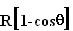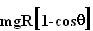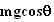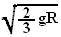## Sunday, October 21, 2007

### Irodov Problem 1.87As shown in the figure, at the time when the mass is at an angleit has descended a height ofand thus has lost a potential energy of. This potential energy will be converted into the mass's kinetic energy and thus we have,The Figure beside shows the forces acting on the mass at some given angle. There are two forces acting on the mass in the radial direction, i) the normal reaction N offered by the surface and ii) the component of force of gravity. The mass also has the centripetal acceleration (note that this becomes the centrifugal force in the reference frame of the observer on the mass and will act radially outwards) given byin the radial direction directed towards the center of the circle.
Thus, we have,When the body is about to leave the surface, the normal reaction becomes N=0. In other words we have,At this angle the velocity of the mass can be calculated using (1) and substituting the obtained value of angle as,.